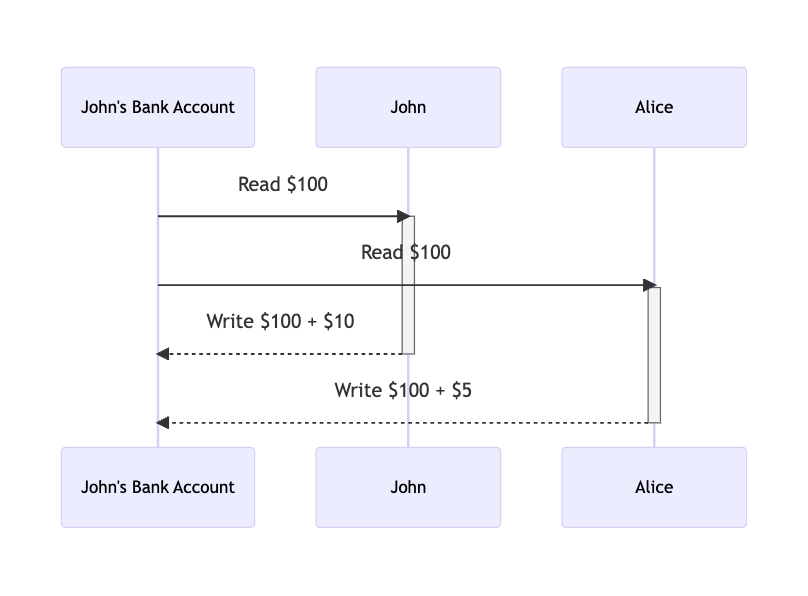# 後端工程師面試考什麼 - Race Condition 篇

Posted on  Oct 17, 2022  in  後端面試準備  by  Amo Chen  ‐ 3 min read

### Race condition / Race hazard #1. 平行處理
2. 對同一個資源同時進行存取操作

``````import threading

JOHN_TOTAL = 0

global JOHN_TOTAL
for _ in range(100000):
JOHN_TOTAL += num

while JOHN_TOTAL == 0:

print(f"John's total: {JOHN_TOTAL}")
``````p.s. 上述範例用 while 迴圈不斷嘗試，直到 race condition 出現為止，可能要試一陣子才會出現，建議可以貼到Google Colab 執行看看，較容易試出 race condition

### Race condition 的解決方法 - Lock #

``````import threading

JOHN_TOTAL = 0

lock.acquire()
global JOHN_TOTAL
for _ in range(100000):
JOHN_TOTAL += num
lock.release()

RETRY_COUNT = 0
while JOHN_TOTAL == 0 and RETRY_COUNT < 10:

print(f"John's total: {JOHN_TOTAL}")
RETRY_COUNT += 1
``````

### 總結 #

Race condition 不單單只存在於多 thread / 多 process 的情況，只要符合以下 2 點，就可能發生：

1. 平行處理
2. 對同一個資源同時進行存取操作

https://en.wikipedia.org/wiki/Race_condition